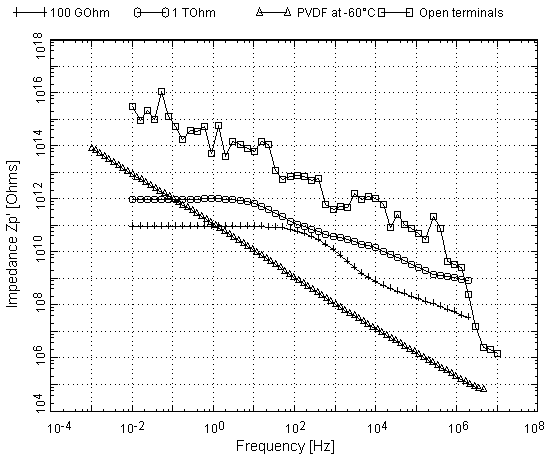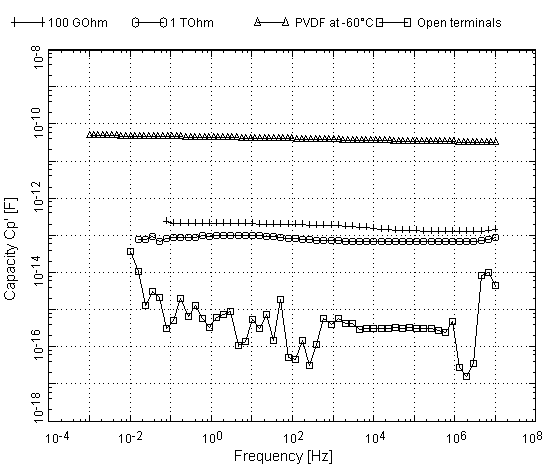Excellence in Broadband Dielectric Spectroscopy • Impedance Spectroscopy • Conductivity Spectroscopy

# Alpha-A Analyzer Measurement Examples:

## High impedance materials and componentsFig. 1. Parallel resistance of two high impedance resistors, a PVDF material sample and the ZG4 test interface high impedance limit (open terminals). Data were measured with an Alpha-ANB mainframe with ZG4 test interface. PVDF data were measured with a ZGS active sample cell test interface.

Some data are shown in terms of parallel resistance Zp' in Fig. 1 and parallel capacity Cp' in Fig. 2.

The crosses and circles show the results for two resistors of 1011 Ω and 1012 Ω. The parallel resistance results Zp' appear flat in the low-frequency regime, showing the nominal values of 1 TΩ and 100 GΩ up to 3 Hz and 30 Hz, respectively. At higher frequencies, the resistance decreases by about three orders of magnitude due to internal dielectric relaxation of the used resistor material.Fig. 2. Parallel capacity of two high impedance resistors, a PVDF material sample and the ZG4 test interface (open terminals) low capacity limit.

For the 100 GΩ and 1 TΩ resistors, the measured capacities are nearly flat, exhibiting values around 200 fF and 100 fF, respectively. The capacity contribution is mainly due to the resistor wires and electrode stray capacities. The small capacity increase to low frequencies of 90 fF for the 100 GΩ resistor and 30 fF for the 1 TΩ resistor is due to the dielectric relaxation of the resistor material and consistent with the Zp' data.

The triangles show data of PVDF at −60 °C, a highly insulating dielectric material. Here the parallel resistance Zp' attains extraordinarily high values, especially in the low-frequency region.

As expected for a dielectric material with comparatively low loss, the capacity is nearly flat over the entire frequency range.

For comparison, both plots show correponding data of the open ZG4 impedance ports. These represent the typical high impedance and low capacity instrumental limits.